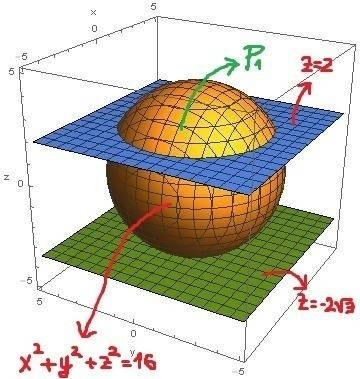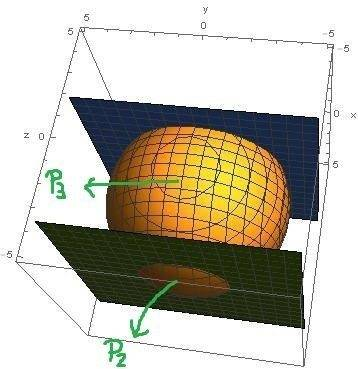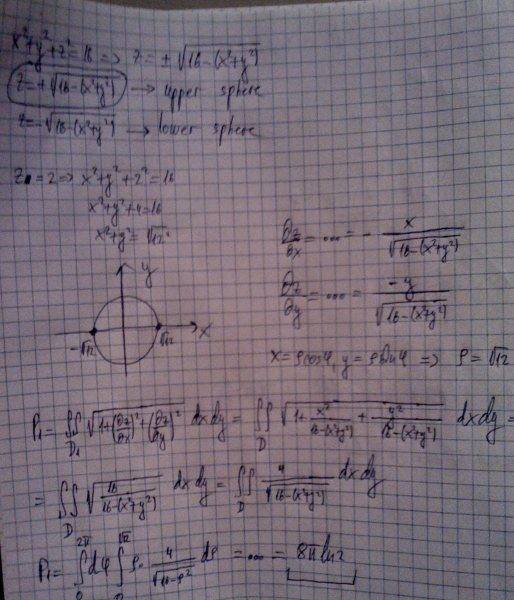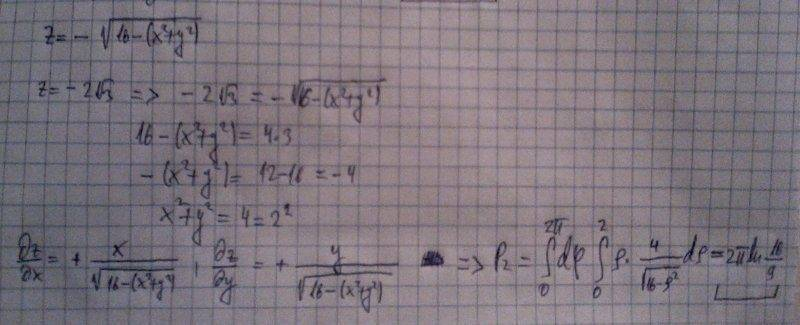# Surface area - Double integrals

Hi!
Calculate surface area of sphere $$x^{2}+y^{2}+z^{2}=16$$ between $$z=2$$ and $$z=-2\sqrt{3}$$.
Here are 3D graphs of our surfaces:Surface area of interest is P3. It would be P-(P1+P2), where P is surface area of whole sphere. Is it correct?
Here is how I calculated P1:Similarly for P2:We calculate P using formula 4pi*r*r.
Is it correct?

SteamKing
Staff Emeritus
Homework Helper

P1=8*pi*ln2, P2=2*pi*ln(16/9), P=4*pi*16
P=4*pi*16-(8*pi*ln2+2*pi*ln(16/9))=201.0619-21.0358=180.0261

SteamKing
Staff Emeritus
Homework Helper
P1=8*pi*ln2, P2=2*pi*ln(16/9), P=4*pi*16
P=4*pi*16-(8*pi*ln2+2*pi*ln(16/9))=201.0619-21.0358=180.0261

http://en.wikipedia.org/wiki/Spherical_segment

It seems there is a disagreement somewhere in your calculations between the formula and your result.

LCKurtz
Homework Helper
Gold Member
etf, have you given any consideration to working the problem in spherical coordinates? You can calculate it directly in about two steps.

I'm not familiar with spherical coordinates :(

STEMucator
Homework Helper
Doing multiple integrals and not being familiar with integral transforms is not a good thing. Polar, spherical and cylindrical co-ordinates are important to be able to integrate properly, and without them, you wont get very far.

An interesting way to do this problem is to describe each face of the surface and then perform a surface integral over each one:

$$\iint_S \space dS = \iint_{S_1} \space dS_1 + \iint_{S_2} \space dS_2 + \iint_{S_3} \space dS_3$$

So you have a flat top surface ##S_1## described by the plane ##z = 2## and bounded by the region ##D_1## given by ##x^2 + y^2 = 12##. You also have a flat bottom surface ##S_2## describe by the plane ##z = -2 \sqrt{3}## and bounded by the region ##D_2##. Finally, you have the spherical middle section bounded by the planes.

SteamKing
Staff Emeritus
Homework Helper
Doing multiple integrals and not being familiar with integral transforms is not a good thing. Polar, spherical and cylindrical co-ordinates are important to be able to integrate properly, and without them, you wont get very far.

An interesting way to do this problem is to describe each face of the surface and then perform a surface integral over each one:

$$\iint_S \space dS = \iint_{S_1} \space dS_1 + \iint_{S_2} \space dS_2 + \iint_{S_3} \space dS_3$$

So you have a flat top surface ##S_1## described by the plane ##z = 2## and bounded by the region ##D_1## given by ##x^2 + y^2 = 12##. You also have a flat bottom surface ##S_2## describe by the plane ##z = -2 \sqrt{3}## and bounded by the region ##D_2##. Finally, you have the spherical middle section bounded by the planes.
I think the OP was supposed to calculate only the area of the spherical surface lying between the planes z = -2√3 and z = 2. I don't think the areas of S1 or S2 were ever intended to be included.

STEMucator
Homework Helper
I think the OP was supposed to calculate only the area of the spherical surface lying between the planes z = -2√3 and z = 2. I don't think the areas of S1 or S2 were ever intended to be included.

Sorry, I saw the problem a little differently when I first read it this morning. The sphere with its ends cut off seems to be more appropriate, so the ##dS_3## integral would be the only thing required. I believe the conventional method of evaluation then proceeds as usual:

$$\iint_S \space dS = \iint_D \sqrt{z_x^2 + z_y^2 + 1} \space dA$$

The two planes and sphere give two circles in the x-y plane from which the limits can be deduced.

LCKurtz
$$\iint_S \space dS = \iint_D \sqrt{z_x^2 + z_y^2 + 1} \space dA$$